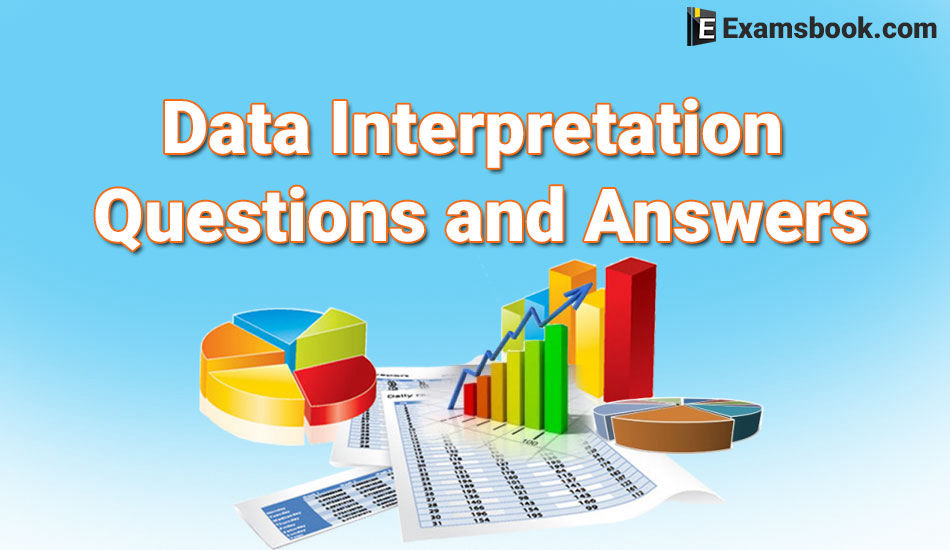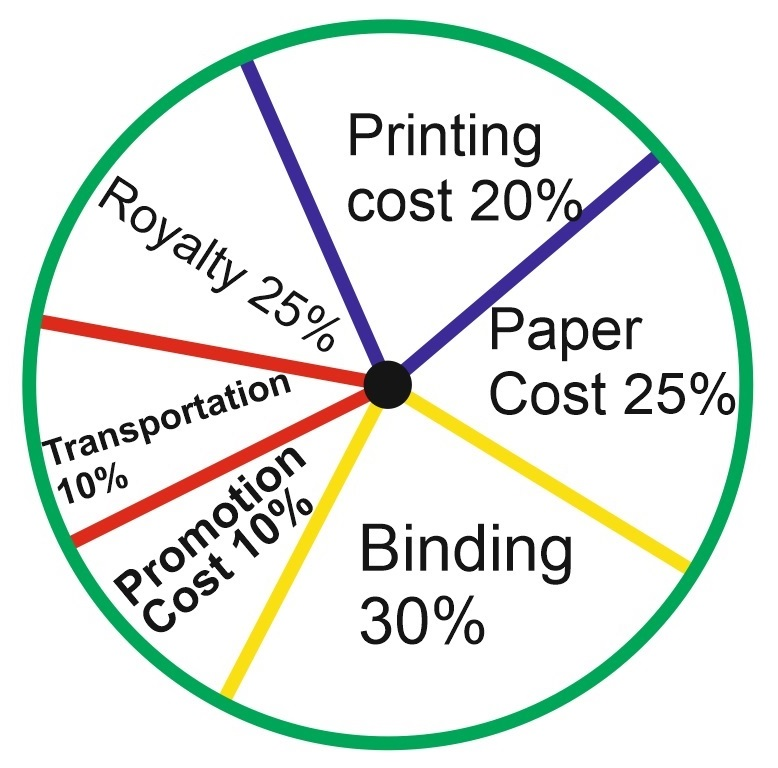• Save

# Data Interpretation Questions and Answers for Bank PO and SSC

3 months ago 3.7K ViewsData interpretation is an essential part of quantitative aptitude. In this data interpretation blog given all the topics like the bar graph, line graph, pie chart, table chart with the data interpretation questions and answers, which are the very important topics for bank exam, SSC or other competitive exams.

If you are preparing for any competitive exams like SSC, IBPS PO, Bank exam, etc. this blog will be very helpful for you. So, You should practice these data interpretation questions with answers to get the good marks in your competitive exams.

## Choose any Data Interpretation Topic for Practice with Questions and Answers

Direction (Question 1 to 9): The following pie-chart shows the percentage distribution of the expenditure incurred in published a book. Study the pie-chart and answer the question based on it.

VARIOUS EXPENDITURES (IN PERCENTAGE) INCURRED IN PUBLISHING A BOOKQ.1. What is the central angle of the sector corresponding to the expenditure incurred on Royalty?

(A) 150

(B) 240

(C) 540

(D) 480

Ans .   C

Q.2. Which two expenditures together have a central angle of 1080?

(A) Binding Cost and Transportation Cost

(B) Printing Cost and Paper Cost

(C) Royalty and Promotion Cost

(D) Binding Cost and Paper Cost

Ans .   A

Q.3. If the difference between the two expenditures is represented by 180 in the pie-chart, then these expenditures possibly are:

(A) Binding Cost and Promotion Cost

(B) Paper Cost and Royalty

(C) Binding Cost and Printing Cost

(D) Paper Cost and Printing Cost

Ans .   D

Q.4. If for an edition of the book, the cost of paper is Rs. 56250, then find the promotion cost for this edition?

(A) Rs. 20,000

(B) Rs. 22,500

(C) Rs. 25,500

(D) Rs. 28,125

Ans .   B

Q.5. If for a certain quantity of books, the publisher has to pay Rs. 30,600 as printing cost, then what will be the amount of royalty to be paid for these books?

(A) Rs. 19,450

(B) Rs. 21,200

(C) Rs. 22,950

(D) Rs. 26,150

Ans .   C

Q.6. The price of the book is marked 20% above the C.P. If the market price of the book is Rs. 180, then what is the cost of the paper used in a single copy of the books?

(A) Rs. 36

(B) Rs. 37.50

(C) Rs. 42

(D) Rs. 44.25

Ans .   B

Q.7. For an edition of 12,500 copies, the amount of Royalty paid by the publisher is Rs. 2,18,250. What should be the selling price of the book if the publisher desires a profit of 5%?

(A) Rs. 152.50

(B) Rs. 157.50

(C) Rs. 162.50

(D) Rs. 167.50

Ans .   B

Q.8. If 5500 copies are published and the transportation cost on them amounts to Rs. 82,500, then what should be the selling price of the book so that the publisher can earn a profit of 25%?

(A) Rs. 187.50

(B) Rs. 191.50

(C) Rs. 175

(D) Rs. 180

Ans .   A

If you face any difficulty in data interpretation questions and answers, you can ask me in comment section anything about data interpretation questions and answers. Go to next page for more practice with data interpretation questions and answers for SSC and Bank Exams.